# Properties of Tangents Drawn to Circles (VC)

## DIRECTIONS:

In the applet below, note the circle with center A. You can alter the radius by dragging the unlabeled point on the circle. Point B is a point on the circle. Point C is a point that lies outside the circle. Feel free to alter the locations of any of these 4 points at any time. 1. Select the TANGENTStool. Then select point B and then select the circle. This will construct a line through B that is tangent to the circle. 2. Use the SEGMENT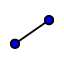tool to construct radius . 3. Use the POINT ON OBJECT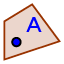tool to plot a point D on this tangent line. Use the ANGLE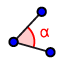tool to measure . (Be sure to select the points in this order.)

4. Select the MOVE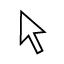tool. Move point B around the circle. What do you notice?

5. Use the TANGENTS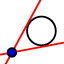tool to construct a line passing through C that is tangent to the circle. How many tangents can be drawn from point C to the circle?

Select all that apply
• A
• B
• C
• D
6. Use the INTERSECTtool to plot the point(s) at which these tangents intersect the circle. GeoGebra should name these points E and F.

7. Use the DISTANCE OR LENGTH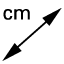tool to measure the distance from C to E and the distance from C to F. What do you notice?

8. What two new properties can we conclude about tangents drawn to circles? Describe.